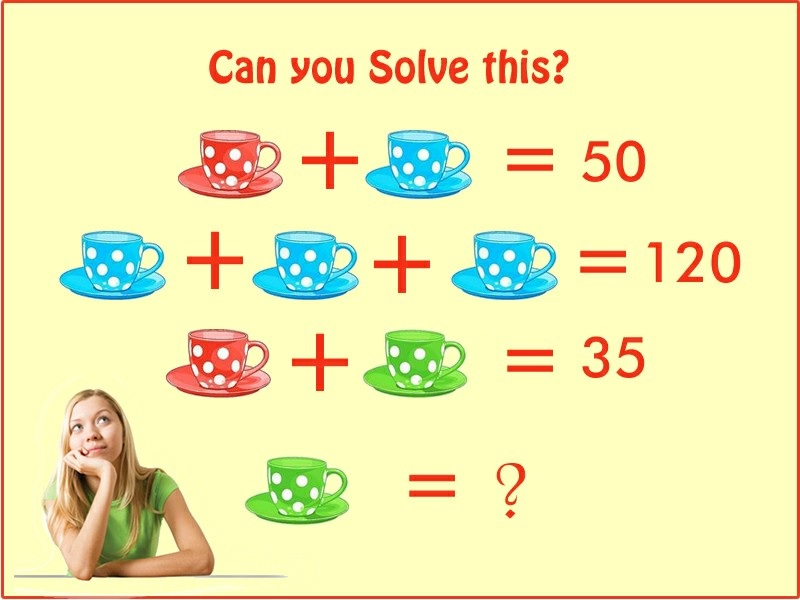This post may contain affiliate links. This means if you click on the link and purchase the item, We will receive an affiliate commission at no extra cost to you. See Our Affiliate Policy for more info.

If Red+Blue Cup=50! Then Find the Value of Green Cup? Genius Math PUZZLES!

0
2099

Math Puzzles and Riddles can boost your Brain. So, Here’s an interesting Fun Brainteaser Puzzles for you. Let’s see if you can solve this. There are 3 equation given,and which is forms by RED, BLUE, GREEN cups.

Solve this equations and  find the value of GREEN CUP.

Can You Solve This?Find the VALUE ofGREEN CUP ?SOLUTION :-

RED CUP + BLUE CUP = 50  …. (EQUATION 1 )

BLUE CUP + BLUE CUP + BLUE CUP = 120    ……(EQUATION 2 )

IF WE, CONSIDER BLUE CUP =

A + A + A = 120

3 A = 120

A = 40

BLUE CUP ( A ) = 40

PUT , A = 40 in equation (1)

RED CUP + 40 = 50

RED CUP = 50 40 = 10

PUT  RED CUP = 10 in equation ( 3 )

RED CUP + GREEN CUP = 35

10 + GREEN CUP = 35

GREEN CUP = 35 – 10

GREEN CUP = 25